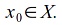## The Fundamental Group of the Space (X,T)

Given a topological space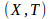and a point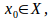let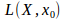be the set of loops in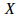with base point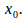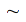is an equivalence relation on a set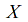for the set of loops with the same base point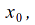with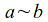if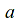is a loop inhomotopic to a loop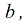andand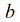have the same base point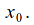The set of homotopy classes is then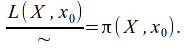The operation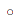of composing loops is associative and has identity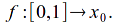Also ifis a loop,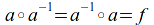and since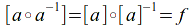the inverse of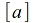is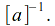The space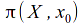with operationis a group with identity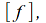called the fundamental group of the spacebased on